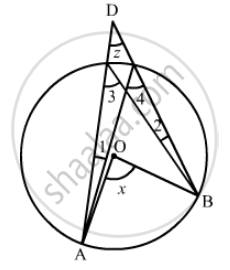Advertisement Remove all ads

# In the given figure, O is the centre of the circle, prove that ∠x = ∠y + ∠z. - Mathematics

Short Note

In the given figure, is the centre of the circle, prove that ∠x = ∠y + ∠z.Advertisement Remove all ads

#### Solution

It is given that, O is the center of circle and AB and C are points on circumference on triangleWe have to prove that x = ∠y + ∠z

∠4
and ∠3 are on same segment

So, ∠4 = ∠3
angle x = 2 angle3  (Angle subtended by an arc of a circle at the centre is double the angle subtended by it at any point on the remaining part of the circle)

anglex = angle 4 + angle 3               …… (1)

angley= angle 3 + angle 1           (Exterior angle is equal to the sum of two opposite interior angles)         …… (2)

$\angle4 = \angle z + \angle1$

(Exterior angle is equal to the sum of two opposite interior angles)

anglez= angle 4 -  angle 1              …… (3)

Adding (2) and (3)

angley + angle z  = angle 3 + angle 4     ……(4)

From equation (1) and (4) we have

anglex = angle y + angle z

Is there an error in this question or solution?
Advertisement Remove all ads

#### APPEARS IN

RD Sharma Mathematics for Class 9
Chapter 15 Circles
Exercise 15.4 | Q 10 | Page 74
Advertisement Remove all ads

#### Video TutorialsVIEW ALL 

Advertisement Remove all ads
Share
Notifications

View all notifications

Forgot password?
Course